# PSEB 7th Class Maths Solutions Chapter 9 Rational Numbers Ex 9.2

Punjab State Board PSEB 7th Class Maths Book Solutions Chapter 9 Rational Numbers Ex 9.2 Textbook Exercise Questions and Answers.

## PSEB Solutions for Class 7 Maths Chapter 9 Rational Numbers Ex 9.2

1. Find the sum

Question (i).
$$\frac{6}{9}+\frac{2}{9}$$
Solution:
$$\frac{6}{9}+\frac{2}{9}$$ = $$\frac{6+2}{9}$$
= $$\frac {8}{9}$$

Question (ii).
$$\frac{-15}{7}+\frac{9}{7}$$
Solution:
$$\frac{-15}{7}+\frac{9}{7}$$ = $$\frac{-15+9}{7}$$
= $$\frac{-6}{7}$$

Question (iii).
$$\frac{17}{11}+\left(\frac{-9}{11}\right)$$
Solution:
$$\frac{17}{11}+\left(\frac{-9}{11}\right)$$ = $$\frac{17-9}{11}$$
= $$\frac{8}{11}$$

Question (iv).
$$\frac{-5}{6}+\frac{3}{18}$$
Solution:
$$\frac{-5}{6}+\frac{3}{18}$$
Now, $$\frac{-5}{6}=\frac{-5}{6} \times \frac{3}{3}=\frac{-15}{18}$$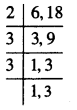L.C.M. of 6 and 18
= 2 × 3 × 3 = 18
Thus, $$\frac{-5}{6}+\frac{3}{18}=\frac{-15}{18}+\frac{3}{18}$$
= $$\frac{-15+3}{18}$$
= $$\frac {-12}{18}$$
= $$\frac {-2}{3}$$Question (v).
$$\frac{-7}{19}+\frac{-3}{38}$$
Solution:
$$\frac{-7}{19}+\frac{-3}{38}$$
Now, $$\frac{-7}{19}=\frac{-7}{19} \times \frac{2}{2}$$
= $$\frac {-14}{38}$$
$$\begin{array}{l|l} 2 & 19,38 \\ \hline 19 & 19,19 \\ \hline & 1,1 \\ \hline \end{array}$$
L.C.M. = 2 × 19
= 38
Thus, $$\frac{-7}{19}+\frac{-3}{38}=\frac{-14}{38}+\frac{-3}{38}$$
= $$\frac{-14-3}{38}$$
= $$\frac{-17}{38}$$

Question (vi).
$$-3 \frac{4}{7}+2 \frac{3}{7}$$
Solution:
$$-3 \frac{4}{7}+2 \frac{3}{7}$$
= $$-\frac{25}{7}+\frac{17}{7}$$
= $$\frac{-25+17}{7}$$
= $$\frac{-8}{7}$$

Question (vii).
$$\frac{-5}{14}+\frac{8}{21}$$
Solution:
$$\frac{-5}{14}+\frac{8}{21}$$
Now, $$\frac{-5}{14}=\frac{-5}{14} \times \frac{3}{3}$$
= $$\frac{-15}{42}$$
$$\begin{array}{l|l} 2 & 14,21 \\ \hline 3 & 7,21 \\ \hline 7 & 7,7 \\ \hline & 1,1 \end{array}$$
L.C.M of 14, 21 = 2 × 3 × 7
= 42
$$\frac{8}{21}=\frac{8}{21} \times \frac{2}{2}$$
= $$\frac{16}{42}$$
Thus, $$\frac{-5}{14}+\frac{8}{21}$$
= $$\frac{-15}{42}+\frac{16}{42}$$
= $$\frac{-15+16}{42}$$
= $$\frac{1}{42}$$

Question (viii).
$$-4 \frac{1}{15}+3 \frac{2}{20}$$
Solution: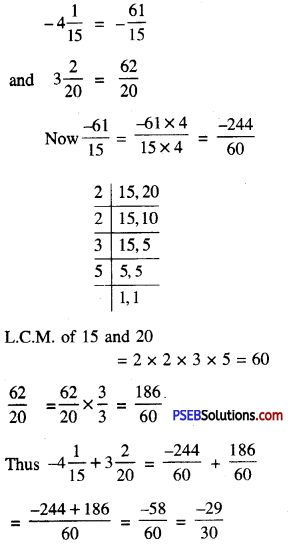2. Find

Question (i).
$$\frac{7}{12}-\frac{11}{36}$$
Solution:
$$\frac{7}{12}-\frac{11}{36}$$ = $$\frac{7}{12}$$ + (Additive inverse of $$\frac{11}{36}$$)
= $$\frac{7}{12}+\left(\frac{-11}{36}\right)$$
= $$\frac{21+(-11)}{36}$$
$$\begin{array}{l|l} 2 & 12,36 \\ \hline 2 & 6,18 \\ \hline 3 & 3,9 \\ \hline 3 & 1,3 \\ \hline & 1,1 \end{array}$$
L.C.M of 12 and 36
= 2 × 2 × 3 × 3
= 36
= $$\frac{10}{36}=\frac{5}{18}$$

Question (ii).
$$\frac{-5}{9}-\frac{3}{5}$$
Solution:
$$\frac{-5}{9}-\frac{3}{5}$$ = $$\frac {-5}{9}$$ + (additive inverse of $$\frac {3}{5}$$)
= $$\frac{-5}{9}+\left(\frac{-3}{5}\right)$$
= $$\frac{-25+(-27)}{45}$$
L.C.M of 9 and 5 is 45 = $$\frac {-52}{45}$$

Question (iii).
$$\frac{-7}{13}-\left(\frac{-5}{91}\right)$$
Solution:
$$\frac{-7}{13}-\left(\frac{-5}{91}\right)$$ = $$\frac {-7}{13}$$ + (additive inverse of $$\frac {-5}{91}$$)
= $$\frac{-7}{13}+\left(\frac{5}{91}\right)$$
= $$\frac{-49+(5)}{91}$$
L.C.M of 13 and 91 = 7 × 13 = 91
$$\begin{array}{l|l} 7 & 13,91 \\ \hline 13 & 13,13 \\ \hline & 1,1 \end{array}$$
= $$\frac {-44}{91}$$Question (iv).
$$\frac{6}{11}-\frac{-3}{4}$$
Solution:
$$\frac{6}{11}-\frac{-3}{4}$$ = $$\frac {6}{11}$$ + (additive inverse of $$\frac {-3}{4}$$)
= $$\frac{6}{11}+\left(\frac{3}{4}\right)$$
= $$\frac{24+33}{44}$$
L.C.M of 11 and 4 is 44 = $$\frac {57}{44}$$

Question (v).
$$3 \frac{4}{9}-\frac{28}{63}$$
Solution: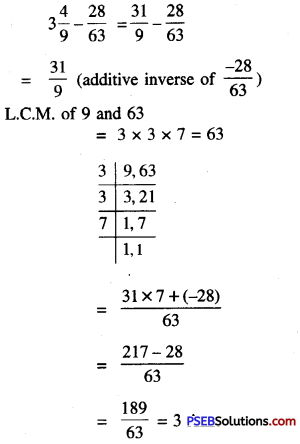3. Find the product of :

Question (i).
$$\frac{5}{9} \times \frac{-3}{8}$$
Solution:
$$\frac{5}{9} \times \frac{-3}{8}$$
= $$\frac{5 \times-3}{9 \times 8}$$
= $$\frac {-5}{24}$$

Question (ii).
$$\frac{-3}{7} \times \frac{7}{-3}$$
Solution:
$$\frac{-3}{7} \times \frac{7}{-3}$$
= $$\frac{-3 \times 7}{7 \times-3}$$
= 1Question (iii).
$$\frac{3}{13} \times \frac{5}{8}$$
Solution:
$$\frac{3}{13} \times \frac{5}{8}$$
= $$\frac{3 \times 5}{13 \times 8}$$
= $$\frac {15}{104}$$

Question (iv).
$$\frac {3}{10}$$ × (-18)
Solution:
$$\frac {3}{10}$$ × (-18)
= $$\frac{3 \times-18}{10}$$
= $$\frac{-27}{5}$$

4. Find the value of:

Question (i).
-9 ÷ $$\frac {3}{5}$$
Solution:
-9 ÷ $$\frac {3}{5}$$
= -9 × (Reciprocal of $$\frac {3}{5}$$)
= -9 × $$\frac {5}{3}$$
= -15

Question (ii).
$$\frac {-4}{7}$$ ÷ 4
Solution:
$$\frac {-4}{7}$$ ÷ 4
= $$\frac {-4}{7}$$ × (Reciprocal of 4)
= $$\frac{-4}{7} \times \frac{1}{4}$$
= $$\frac {-1}{7}$$Question (iii).
$$\frac{7}{18} \div \frac{5}{6}$$
Solution:
$$\frac{7}{18} \div \frac{5}{6}$$
= $$\frac {7}{18}$$ × (Reciprocal of $$\frac {5}{6}$$)
= $$\frac{7}{18} \times \frac{6}{5}$$
= $$\frac {7}{15}$$

Question (iv).
$$\frac{-8}{35} \div\left(\frac{-2}{7}\right)$$
Solution:
$$\frac{-8}{35} \div\left(\frac{-2}{7}\right)$$
= $$\frac {-8}{35}$$ × (Reciprocal of $$\frac {-2}{7}$$)
= $$\frac{-8}{35} \times \frac{7}{-2}$$
= $$\frac {4}{5}$$

Question (v).
$$\frac {-9}{15}$$ ÷ -18
Solution:
$$\frac {-9}{15}$$ ÷ -18
= $$\frac {-9}{15}$$ × (Reciprocal of -18)
= $$\frac{-9}{15} \times \frac{1}{-18}$$
= $$\frac {1}{30}$$

5. What ratonal number should be added to $$\frac {-5}{12}$$ to get $$\frac {-7}{8}$$?
Solution:
Let the required number to be added be x.
then, $$\frac {-5}{12}$$ + x = $$\frac {-7}{8}$$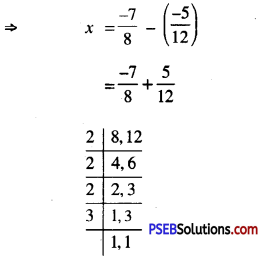L.C.M of 8, 12 = 2 × 2 × 2 × 3
= 24
= $$\frac{-7 \times 3+5 \times 2}{24}$$
= $$\frac{-21+10}{24}$$
= $$\frac {-11}{24}$$6. What number should be subtracted from $$\frac {-2}{3}$$ to get $$\frac {-5}{6}$$ ?
Solution:
Let the required number to be subtracted be x, then
$$\frac{-2}{3}-x=\frac{-5}{6}$$
⇒ $$\frac{-2}{3}-\left(\frac{-5}{6}\right)$$ = x
x = $$\frac{-2}{3}+\frac{5}{6}$$
= $$\frac{-4+5}{6}$$
= $$\frac {1}{6}$$

7. The product of two rational numbers is $$\frac {-11}{2}$$. If one of them is $$\frac {33}{8}$$, find the other number.
Solution:
Let the required number be x, then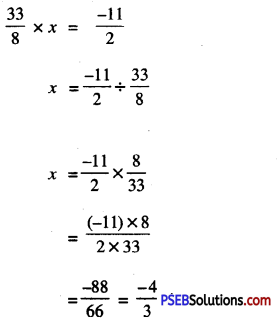8. Multiple Choice Questions

Question (i).
The sum of $$\frac{5}{4}+\left(\frac{25}{-4}\right)$$ =
(a) -5
(b) 5
(c) 4
(d) -4
(a) -5

Question (ii).
$$\frac{17}{11}-\frac{6}{11}$$ =
(a) 1
(b) -1
(c) 6
(d) 3
(a) 1Question (iii).
$$\frac{2}{-5} \times \frac{-5}{2}$$ =
(a) 1
(b) -1
(c) 2
(d) -5
(a) 1

Question (iv).
$$\frac{7}{12} \div\left(\frac{-7}{12}\right)$$ =
(a) 1
(b) -1
(c) 7
(d) -7